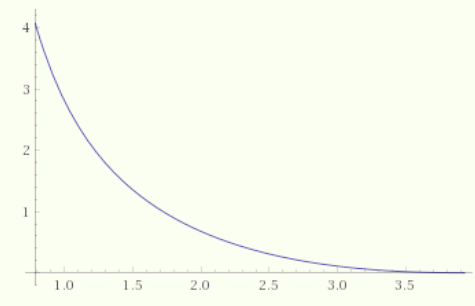# Doubt on maxima and minima

If \color{brown}\displaystyle f(x)=\frac{\sin x+\cos x+\sqrt{2}}{x\sqrt{x}} for all \color{brown}\displaystyle x\in\bigg[\frac{\pi}{4},\frac{5\pi}{4}\bigg].
If m and M be \min and \max value of f(x). Then \displaystyle \bigg\lfloor \frac{2M}{5m}\bigg\rfloor.

Decrease in given interval ,minimum zero

1 Like

Matlab ans infinite. Dont think so

Yahi to dar hai ,

Doesn't exist